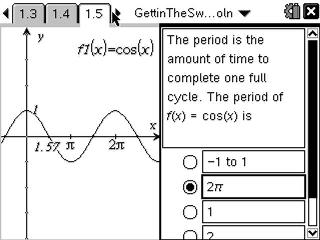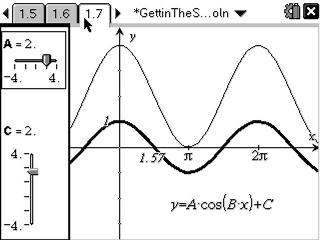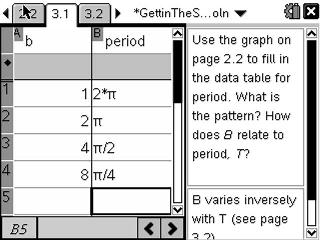# Activities

••• ##### Subject Area

• Math: Calculus: Transcendental Functions
• Math: Calculus: Applications of the Derivative
• Math: Calculus: Derivatives
• Math: Calculus: Other Functions

• ##### Author9-12

45 Minutes

• ##### Device
• TI-Nspire™ Navigator™
• TI-Nspire™
• TI-Nspire™ CAS
• ##### Software

TI-Nspire™
TI-Nspire™ CAS

2.0

CBR™/CBR 2™

• ##### Other Materials
pendulum (smooth ball hanging from a string), meter stick and stop watch

## Gettin' the Swing#### Activity Overview

In this activity, students will investigate sinusoidal functions, collect data from a swinging pendulum, model the data with a cosine function, and take the derivative to find the velocity and acceleration. Self-check multiple-choice questions help students review and build the foundation for their data collection and mathematical model activity.

#### Key Steps

•Students begin the activity by reviewing the range, period, and amplitude of a sinusoidal function using self-check multiple-choice questions.

•Students will then explore sinusoidal functions and the effect of the parameters using sliders. They will continue to answer multiple choice questions to solidify their understand of A, B, C, and D in the equation y = Acos(B(xD)) + C.

•Use of a slider, spreadsheet, and a graph on a Data & Statistics page help students conceptually derive the relationship between B and the period.